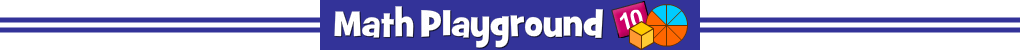Solve multiplication and division problems then go after
the ghost that matches the correct answer.
Mathman Multiplication - Learning Connections
Essential Skills
Mental Math - multiply and divide numbers by memory or through other efficient methods

Common Core Connection for Grades 3 and 4
Know from memory all products of two one-digit numbers.
Apply properties of operations as strategies to multiply and divide.
Fluently divide within 100 using strategies and properties of operations.
More Math Games to Play